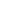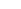# Catenation of Regular Grammars

## Algorithm

For two regular grammar G1 and G2, we construct a regular grammar G such that L(G) = L(G1) L(G2):

1. rename the non-terminals of G1 and G2, so as to have disjoint non-terminal sets. One possible way is to add 1 to the end all the non-terminals in G1, and 2 to those in G2;
2. ifis in L1, modify G1 so as to remove it (hence obtaining an-free grammar);
3. for every rule in G1 of the form Aa, we replace it with the rule AaS2, where S2 is the start symbol of G2.
4. copy all the production from G2 to G1;
5. ifwas in L1, add the rule S1, where S1 is the start symbol in G1.

## Example

Give a regular language recognising the catenation of the languages recognised by the following two grammars:

 SaA | bB SaA | bS AaA | a Aa BbB | b |where S is the start symbol where S is the start symbol

1. Rename the non-terminals:

 S1aA1 | bB1 S2aA2 | bS2 A1aA1 | a A2a B1bB1 | b |where S1 is the start symbol where S2 is the start symbol

2. Removefrom G1 to obtain G:

 S1aA1 | bB1 | b A1aA1 | a B1bB1 | b

where S1 is the start symbol

3. For every rule of the form Aa in G', we replace it with the rule AaS2:

 S1aA1 | bB1 | bS2 A1aA1 | aS2 B1bB1 | bS2

where S1 is the start symbol

4. Now we copy all the rules from G2 to G':

 S1aA1 | bB1 | bS2 A1aA1 | aS2 B1bB1 | bS2 S2aA2 | bS2 A2a

where S1 is the start symbol

5. Since S1was in the language of G1, we addto the language recognised by G':

 SaA1 | bB1 | bS2 |S1aA1 | bB1 | bS2 A1aA1 | aS2 B1bB1 | bS2 S2aA2 | bS2 A2a

where S is the start symbol

## Exercises

Construct a regular grammar that recognises the catenation of the following pairs of languages (described using regular grammars):

1.  SaA | bC SaA | bS AaA | aB AaS | b BbB | b CcB | cC where S is the start symbol where S is the start symbol

2.  SaA | bC SaA | bS AaA | aB Aa BbB | b CcB | cC |where S is the start symbol where S is the start symbol

3.  SaA | bS SaA | bC |Aa AaA | aB BbB | b |CcB | cC where S is the start symbol where S is the start symbol

4.  SaA | bC |SAaA | aB BbB | b |CcB | cC where S is the start symbol where S is the start symbol